Ex 6.5

Chapter 6 Class 10 Triangles (Term 1)
Serial order wise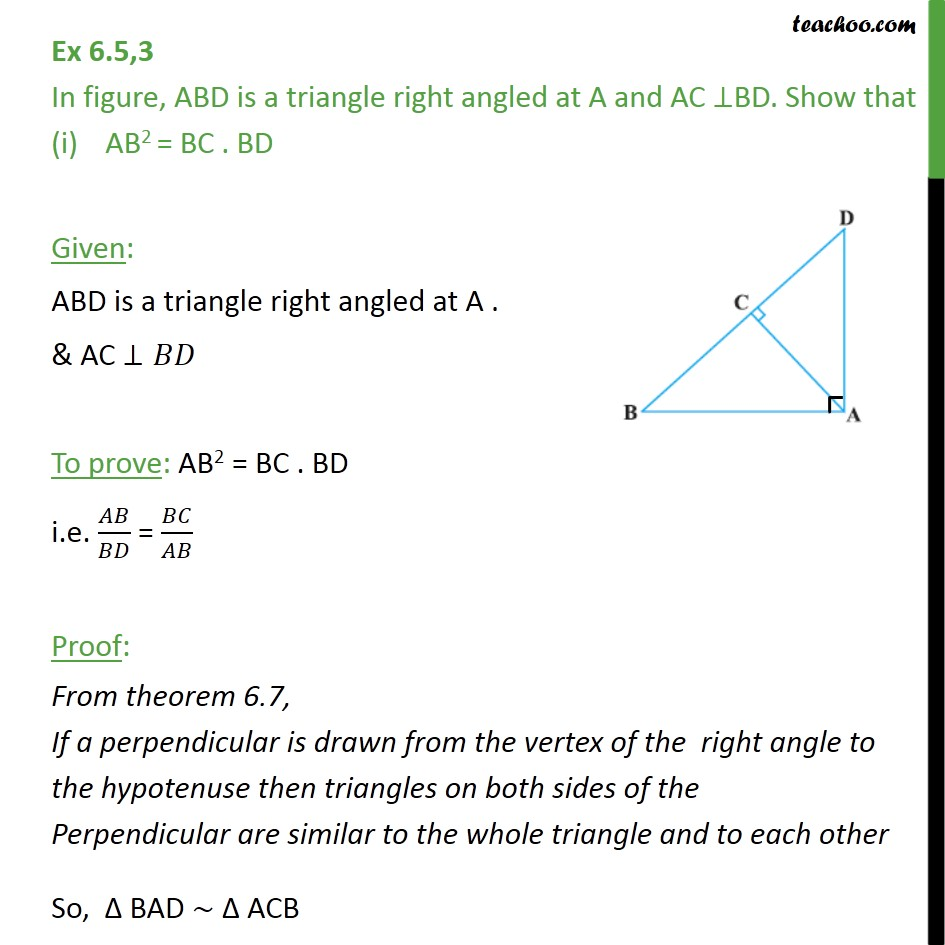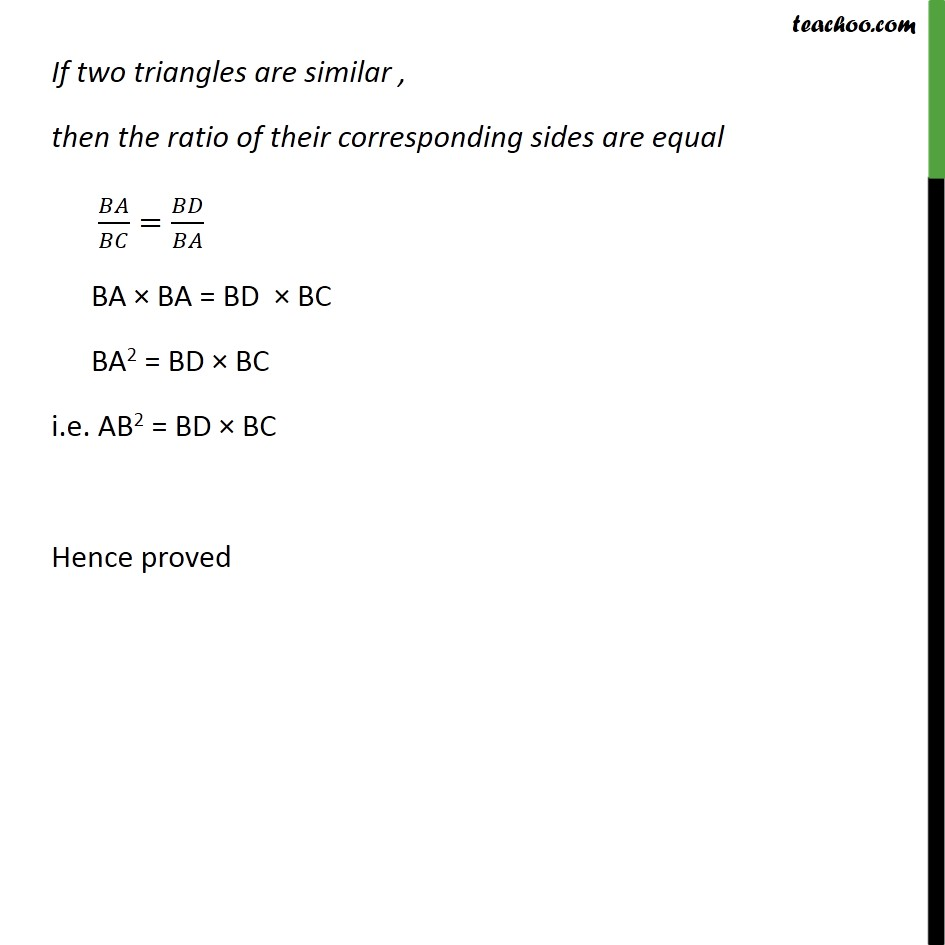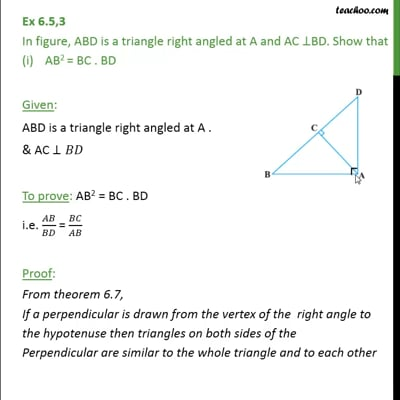This video is only available for Teachoo black users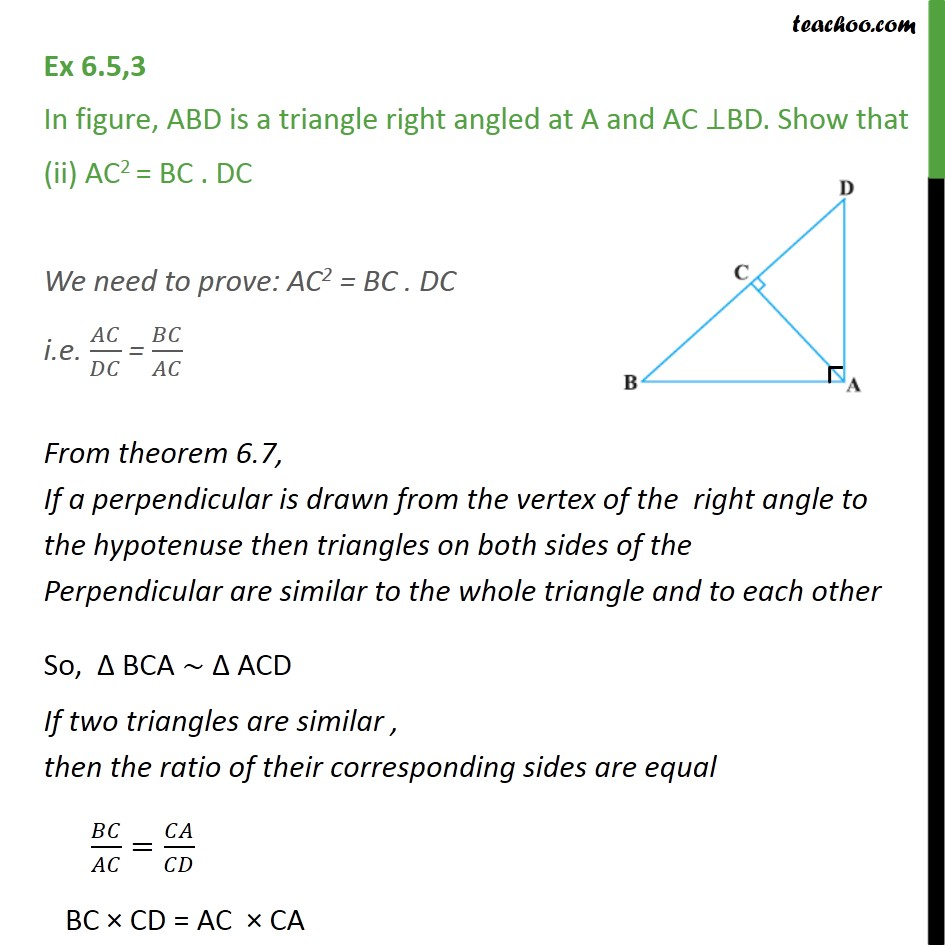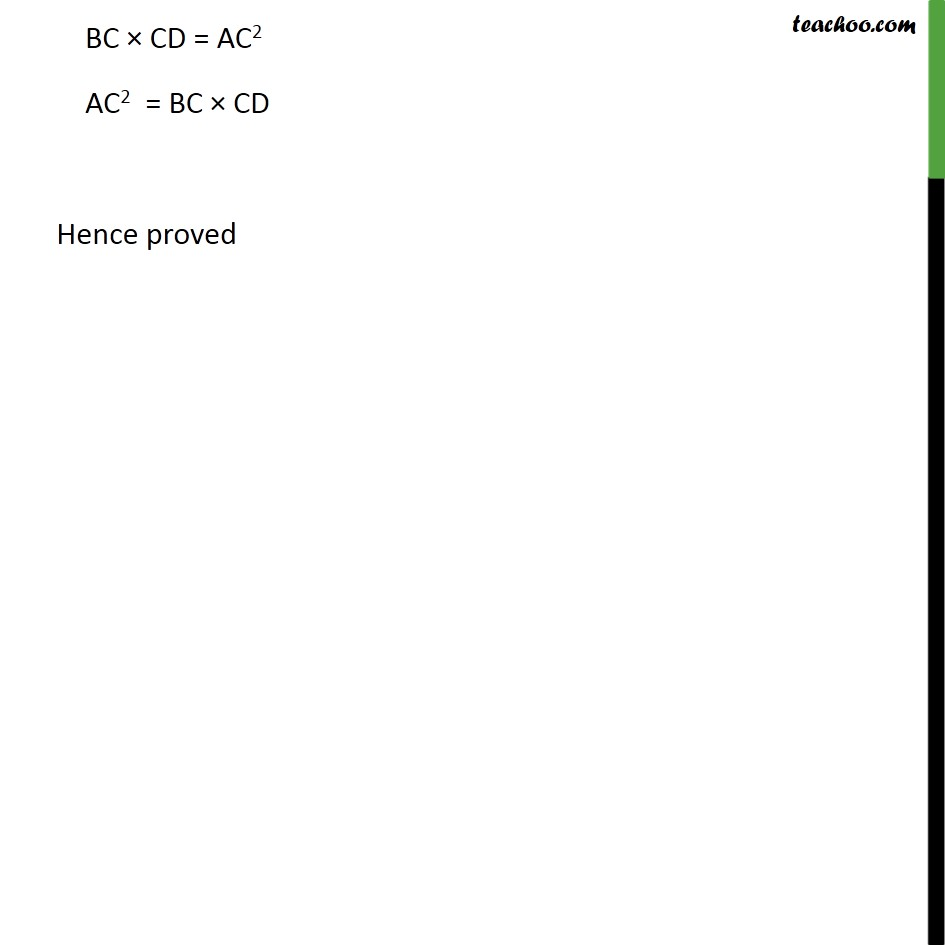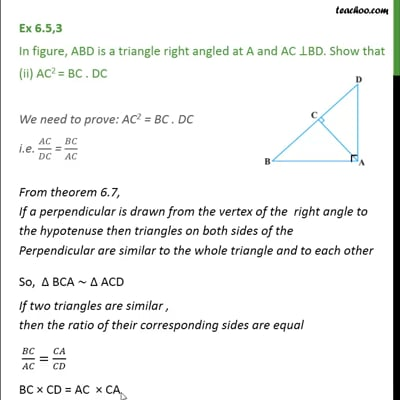This video is only available for Teachoo black users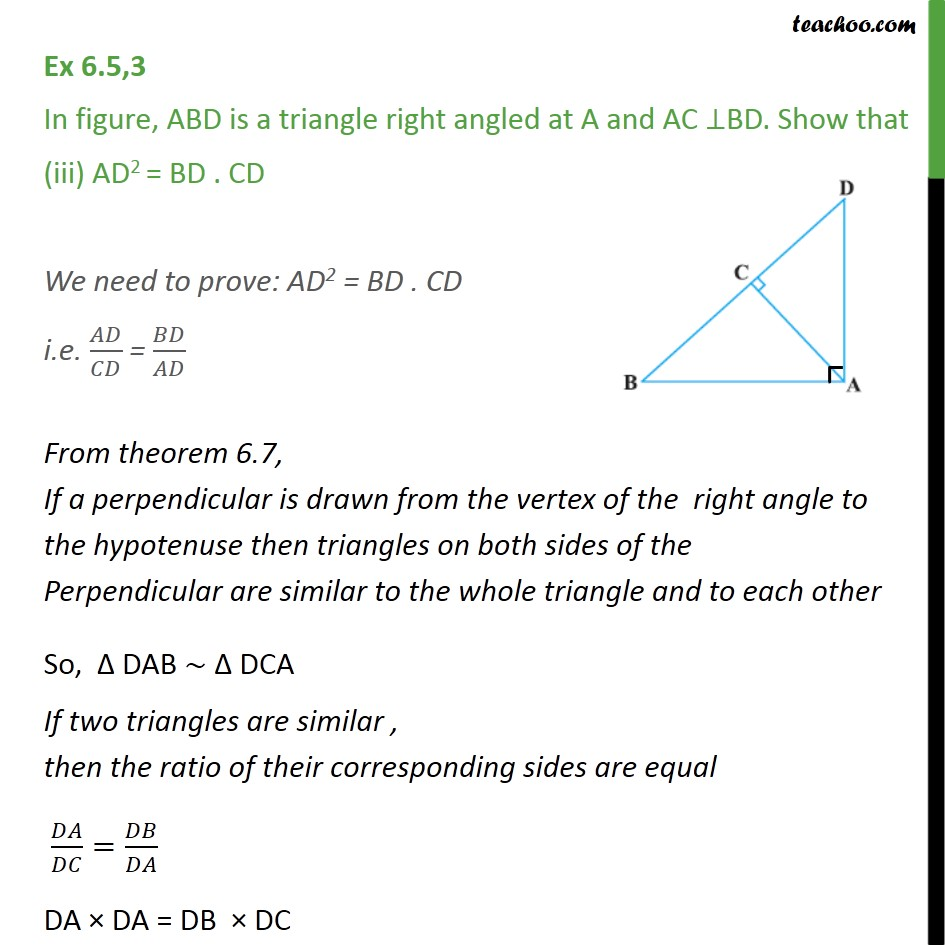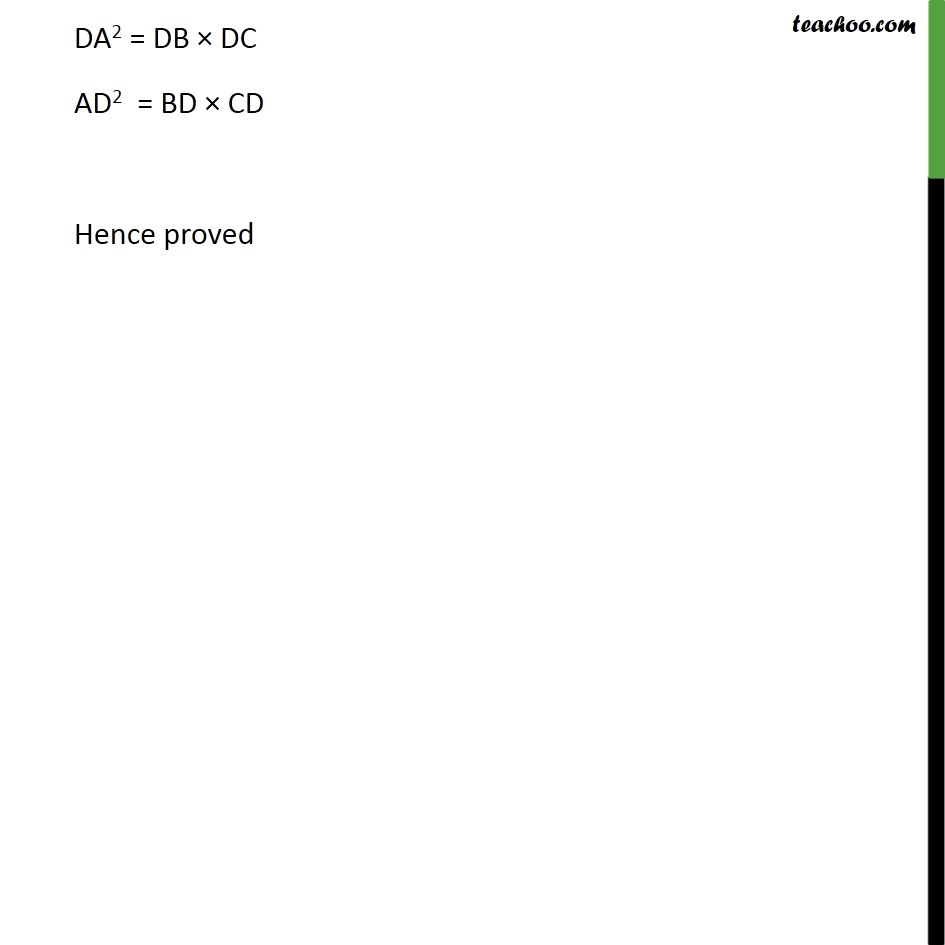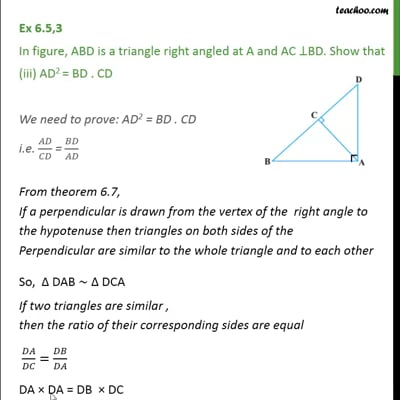This video is only available for Teachoo black users

### Transcript

Ex 6.5,3 In figure, ABD is a triangle right angled at A and AC ⊥ BD. Show that AB2 = BC . BD Given: ABD is a triangle right angled at A . & AC ⊥ 𝐵𝐷 To prove: AB2 = BC . BD i.e. 𝐴𝐵/𝐵𝐷 = 𝐵𝐶/𝐴𝐵 Proof: From theorem 6.7, If a perpendicular is drawn from the vertex of the right angle to the hypotenuse then triangles on both sides of the Perpendicular are similar to the whole triangle and to each other So, Δ BAD ∼ Δ ACB If two triangles are similar , then the ratio of their corresponding sides are equal 𝐵𝐴/𝐵𝐶=𝐵𝐷/𝐵𝐴 BA × BA = BD × BC BA2 = BD × BC i.e. AB2 = BD × BC Hence proved Ex 6.5,3 In figure, ABD is a triangle right angled at A and AC ⊥BD. Show that (ii) AC2 = BC . DC We need to prove: AC2 = BC . DC i.e. 𝐴𝐶/𝐷𝐶 = 𝐵𝐶/𝐴𝐶 From theorem 6.7, If a perpendicular is drawn from the vertex of the right angle to the hypotenuse then triangles on both sides of the Perpendicular are similar to the whole triangle and to each other So, Δ BCA ∼ Δ ACD If two triangles are similar , then the ratio of their corresponding sides are equal 𝐵𝐶/𝐴𝐶=𝐶𝐴/𝐶𝐷 BC × CD = AC × CA BC × CD = AC2 AC2 = BC × CD Hence proved Ex 6.5,3 In figure, ABD is a triangle right angled at A and AC ⊥BD. Show that (iii) AD2 = BD . CD We need to prove: AD2 = BD . CD i.e. 𝐴𝐷/𝐶𝐷 = 𝐵𝐷/𝐴𝐷 From theorem 6.7, If a perpendicular is drawn from the vertex of the right angle to the hypotenuse then triangles on both sides of the Perpendicular are similar to the whole triangle and to each other So, Δ DAB ∼ Δ DCA If two triangles are similar , then the ratio of their corresponding sides are equal 𝐷𝐴/𝐷𝐶=𝐷𝐵/𝐷𝐴 DA × DA = DB × DC DA2 = DB × DC AD2 = BD × CD Hence proved